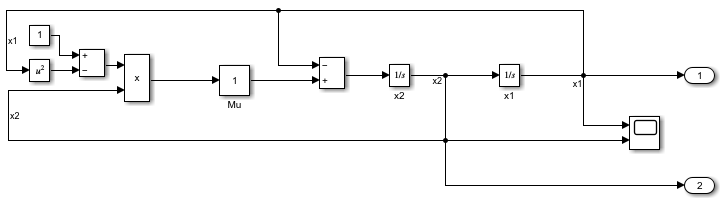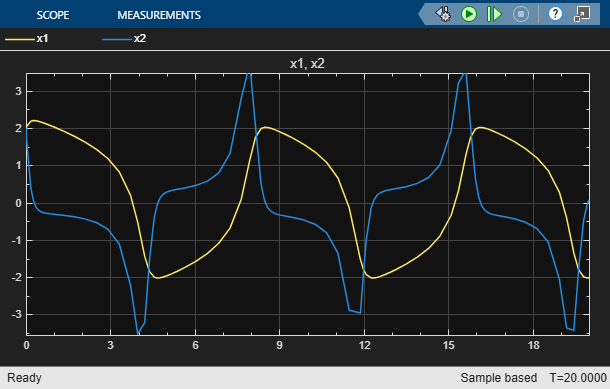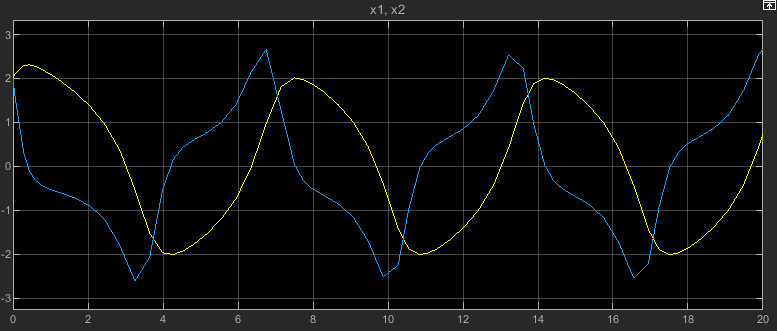Get initial state data from block diagram

## Syntax

``x0 = Simulink.BlockDiagram.getInitialState(mdlName)``

## Description

example

````x0 = Simulink.BlockDiagram.getInitialState(mdlName)` compiles the model specified by `mdlName` and returns the initial values for block states in the model, including states for blocks inside referenced models. If the Format parameter value for the model is `Dataset`, then `x0` is a `Simulink.SimulationData.Dataset` object that contains the initial state information for blocks in the model.If the Format parameter for the model is anything other than `Dataset`, then `x0` is a structure that contains the initial state information for blocks in the model. You can use this initial state information to specify initial block state values for simulation or to provide an initial condition for linearization. You can use the state information as-is, or you can modify individual state values or remove state values from the structure if you want to specify state information for a subset of block states.To simulate a model from an initial simulation state or operating point, consider using a `Simulink.op.ModelOperatingPoint` object instead. The `ModelOperatingPoint` object contains complete information about the model operating point, including block states, hidden block states, the state of the solver and execution engine, and output values for some blocks. For more information, see Specify Initial State for Simulation.```

## Examples

collapse all

Open the model `vdp`. The model uses two Integrator blocks.

```mdl = "vdp"; open_system(mdl)```The Format parameter for the model specifies whether the `Simulink.BlockDiagram.getInitialState` function returns a structure or a `Simulink.SimulationData.Dataset` object. Set the parameter value to `Dataset`.

1. In the Simulink® Toolstrip, on the Modeling tab, click Model Settings.

2. In the Configuration Parameters dialog box, select the Data Import/Export pane.

3. From the Format list, select `Dataset`.

4. In the Configuration Parameters dialog box, click OK.

Alternatively, use the `set_param` function to specify the value for the `SaveFormat` parameter.

`set_param(mdl,"SaveFormat","Dataset")`

Get the `Dataset` object of block states for the model using the `Simulink.BlockDiagram.getInitialState` function.

`initStates = Simulink.BlockDiagram.getInitialState(mdl)`
```initStates = Simulink.SimulationData.Dataset 'xFinal' with 2 elements Name BlockPath ____ _________ 1 [1x1 State] '' vdp/x1 2 [1x1 State] '' vdp/x2 - Use braces { } to access, modify, or add elements using index. ```

Set the value of the state for the Integrator block named `x2` to `2`.

`initStates{2}.Values.Data = 2;`

Remove the element with the state information for the Integrator block named `x1`.

`initStates = removeElement(initStates,1);`

Specify the variable `initStates`, which includes an initial state value for the Integrator block named `x2` as the initial state for the model.

1. In the Simulink Toolstrip, on the Modeling tab, click Model Settings.

2. In the Configuration Parameters dialog box, select the Data Import/Export pane.

3. Select Initial state. Then, in the box, enter `initStates`.

4. Click OK.

Alternatively, use the `set_param` function to configure the `LoadInitialState` and `InitialState` parameters.

`set_param(mdl,"LoadInitialState","on","InitialState","initStates")`

Simulate the model.

`out = sim(mdl);`

To view the output signals for both Integrator blocks, double-click the Scope block. For both Integrator blocks, the initial output value and initial state value are both `2`.For the Integrator block named `x2`, the initial state value of `2` comes from the value of the Initial state parameter. For the Integrator block named `x1`, the initial state value of `2` is specified in the block parameters.

```blk = strcat(mdl,"/x1"); get_param(blk,"InitialCondition")```
```ans = '2' ```

Open the model `vdp`. The model uses two Integrator blocks.

```mdl = "vdp"; open_system(mdl)```The Format parameter for the model specifies whether the `Simulink.BlockDiagram.getInitialState` function returns a structure or a `Simulink.SimulationData.Dataset` object. Set the parameter value to `Structure`.

1. In the Simulink® Toolstrip, on the Modeling tab, click Model Settings.

2. In the Configuration Parameters dialog box, select the Data Import/Export pane.

3. From the Format list, select `Structure`.

4. In the Configuration Parameters dialog box, click OK.

Alternatively, use the `set_param` function to specify the value for the `SaveFormat` parameter.

`set_param(mdl,"SaveFormat","Structure")`

Get the structure of block states for the model using the `Simulink.BlockDiagram.getInitialState` function. The structure contains information for two block states in the `signals` field, one for each Integrator block.

`initStates = Simulink.BlockDiagram.getInitialState(mdl)`
```initStates = struct with fields: time: 0 signals: [1x2 struct] ```

The `signals` structure for each state contains information about the state, including the initial value currently used in the model and the block path.

`initStates.signals(2)`
```ans = struct with fields: values: 0 dimensions: 1 label: 'CSTATE' blockName: 'vdp/x2' stateName: '' inReferencedModel: 0 sampleTime: [0 0] ```

The second block state corresponds to the Integrator block named `x2`. Set the `values` field to `2`.

`initStates.signals(2).values = 2;`

Remove the structure in the `signals` field that includes information about the state for the Integrator block named `x1`.

`initStates.signals(1) = [];`

Specify the variable `initStates`, which includes an initial state value for the Integrator block named `x2` as the initial state for the model.

1. In the Simulink Toolstrip, on the Modeling tab, click Model Settings.

2. In the Configuration Parameters dialog box, select the Data Import/Export pane.

3. Select Initial state. Then, in the box, enter `initStates`.

4. Click OK.

Alternatively, use the `set_param` function to configure the `LoadInitialState` and `InitialState` parameters.

`set_param(mdl,"LoadInitialState","on","InitialState","initStates")`

Simulate the model.

`out = sim(mdl);`

To view the output signals for both Integrator blocks, double-click the Scope block. The initial output value for the Integrator block is the initial state value. For both Integrator blocks, the initial output value and initial state value are both `2`.For the Integrator block named `x2`, the initial state value of `2` comes from the value of the Initial state parameter. For the Integrator block named `x1`, the initial state value of `2` is specified in the block parameters.

```blk = strcat(mdl,"/x1"); get_param(blk,"InitialCondition")```
```ans = '2' ```

## Input Arguments

collapse all

Model name, specified as a string or a character vector.

Data Types: `char` | `string`

## Output Arguments

collapse all

Initial values for block states, returned as a `Simulink.SimulationData.Dataset` object or as a structure.

When the Format parameter value for the model is `Dataset`, the initial state values are returned as a `Dataset` object.

Otherwise, the initial state values are returned as a structure that contains these fields:

• `time` — Simulation time

• `signals``1`-by-n array of structures, where n is the number of block states in the model

Each structure in the `signals` field represents a block state and contains these fields:

• `values` — State value

• `dimensions` — Dimensions of state value

• `label` — State label

For continuous states, the state label is `CSTATE`. The name of the discrete state is shown for discrete states in S-Function blocks and for blocks that assign names to discrete states. For unnamed discrete states, the state label is `DSTATE`.

• `blockName` — Full path to block associated with state

• `inReferencedModel` — Logical indication of whether the state is associated with a block in a referenced model

A value of `1` or `true` indicates that the state is associated with a block in a referenced model. A value of `0` or `false` indicates that the state is associated with a block in the top model.

• `sampleTime` — Sample time information for the block associated with the state

The value is a `1`-by-`2` array where the first element indicates the sample time and the second element indicates the sample time offset.

## Version History

Introduced in R2006b